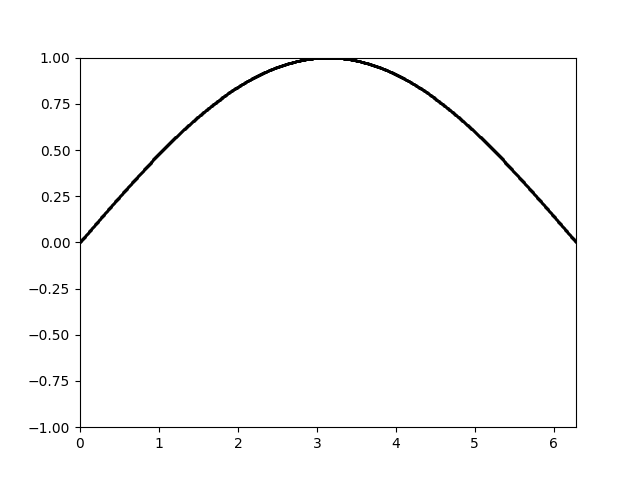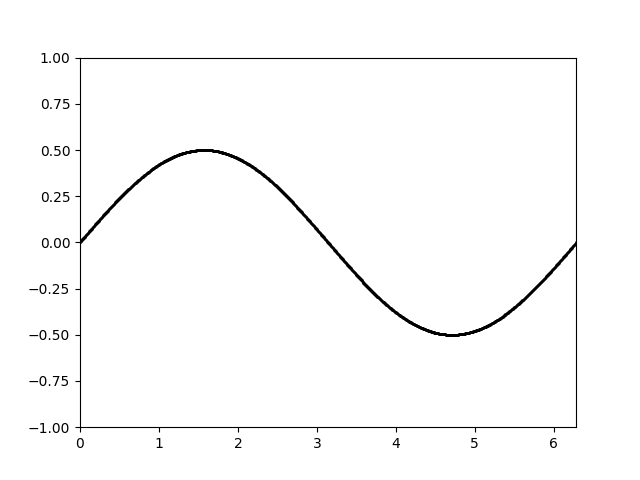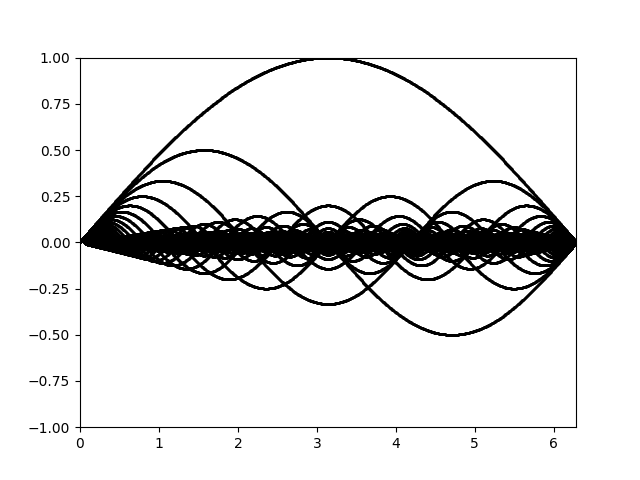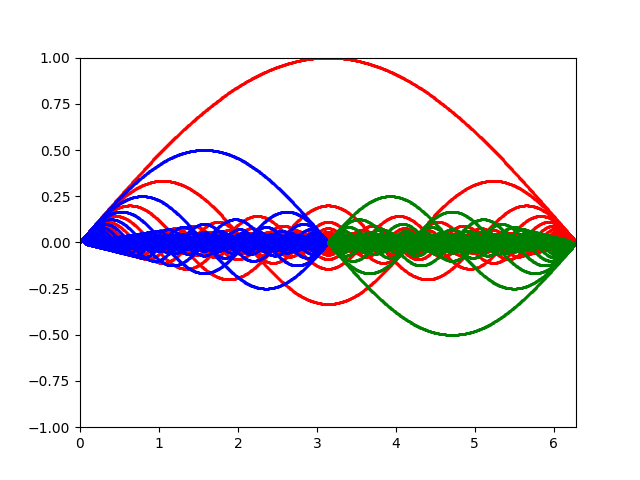# Fractal Harmonic Waves

post by lsusr · 2020-05-31T01:31:35.842Z · score: 10 (7 votes) · LW · GW · 2 comments

This is a link post for https://www.lsusr.com/blog/fractal-harmonic-waves.html

## Contents

  Abstract
Harmonic Waves
Attenuation
Information Density
Self-similarity
Conclusion
Neuroscience
Artificial Intelligence
None


# Abstract

Connectome-Specific Harmonic Waves (CSHWs) [LW · GW] are fractal. I mean this in the strict mathematical sense a self-similar pattern whose fractal dimension exceeds its topological dimension.

In the human brain, "topological dimension" refers to one of the 3 spatial dimensions. In the context of harmonic waves, "fractal dimension" refers to how the brain's information content scales faster than its spatial dimension.

In a digital computer, topological dimension scales equally with spatial dimension. If you double the length of an array then that doubles the number of bits which doubles the information contained. Doubling a topological dimension doubles the information contained.

With harmonic waves, doubling a single topological dimension quadruples the information content. An oscillating brain has fractal dimension 2. Doubling the linear dimensions of a brain increases its information content by a factor of 64.

# Harmonic Waves

CSHWs are the resonant frequencies of the connectome. Consider a 1-dimensional model of the human brain of length . The first harmonic is the fundamental frequency with 1 antinode and wavelength .The second harmonic is the standing wave with 2 antinodes and wavelength .Here are the first 100 harmonics all in phase with each other.The instantaneous state of any wave, including brainwaves, can be approximated as the unique superposition of harmonic waves at various phases and amplitudes.

# Attenuation

It might look like the information density of the superposition of wave states is equals to bits where is the number of harmonics. Two facts complicate this.

First of all, each harmonic has an amplitude and a phase, both selected from a continuous range. This results in a complex Cartesian product containing bits of measurable information instead of single binary bit. This constant doesn't matter for the purposes of this article so I will set .

Secondly and more importantly, different wavelengths propagate different distances.

If we naïvely borrow the attenuation constant from telecommunications we find that wavelength is directly proportional to attenuation constant.

Permittivity and loss tangent can be considered constants.

We can plug this into the amplitude ratio equation.

Total propagation distance is the maximum distance at which a signal can be detected. Let's call this distance . We want to calculate from the minimum detectable amplitude of a wave. (Note: The following equations assume equal peak amplitude for each harmonic, unlike the animations, which assume equal energy.)

We conclude that detectable propagation distance is directly proportional to wavelength .

Suppose for some particular harmonic that . It follows that for all values of . To simplify the math hereon, I will let . Thus .

# Information Density

Due to attenuation, the information content of a harmonic is directly proportional to its frequency. Higher frequency harmonics contain more information and vice-versa. Suppose as before with that the fundamental frequency (first harmonic) contains 1 bit. Then the second harmonic contains 2 bits, the third harmonic contains 3 bits and so on. The total information of a brain's waves is the sum of the information contained in all of its harmonics.

. Therefore .

Remember .

Information represented by a harmonic connectome scales at in each spatial dimension instead of . Its fractal dimension equals 2.

# Self-similarity

We have shown that the information content of the human brain has fractal dimension 2. Now we can explore the self-similarity of harmonic waves.

I've color-coded the hundred harmonics animation from earlier to show how the Fourier decomposition contains two smaller copies of itself. These copies are colored blue and green.If the blue and green mini copies were all the original decomposition contained then harmonic waves would have fractal dimension 1. The extra bandwidth comes from the the red waves. The red waves have a information content equal to the blue and green waves combined.

The red waves are not so visually self-similar to the blue and green [LW · GW] waves, but do make conceptual mathematical sense if you consider odd and even functions as belonging to SU(2).

# Conclusion

The fractal structure of harmonic waves could have big implications for neuroscience and potentially even bigger implications for artificial intelligence.

## Neuroscience

The biggest problem with the CSHW theory is that harmonic waves don't contain enough information. At least, that would be a problem if they had fractal dimension 1. But harmonic waves have fractal dimension 2 and the human brain has 3 spatial dimensions. Doubling the linear dimensions of a brain increases its information content rate by a factor of .

## Artificial Intelligence

The biggest challenge in artificial intelligence is we don't know how [LW · GW] to generalize from high-frequency short-term big data to low-frequency long-term small data. Structuring a machine fractally might be the first step to inventing a true artificial general intelligence.

1. Technically-speaking, CSHW refers to "eigendecomposition of the Laplace operator", to which Fourier decomposition is a special case. In this paper, I treat Laplace eigendecomposition and Fourier decomposition as if they were the same thing in order to simplify the math. ↩︎